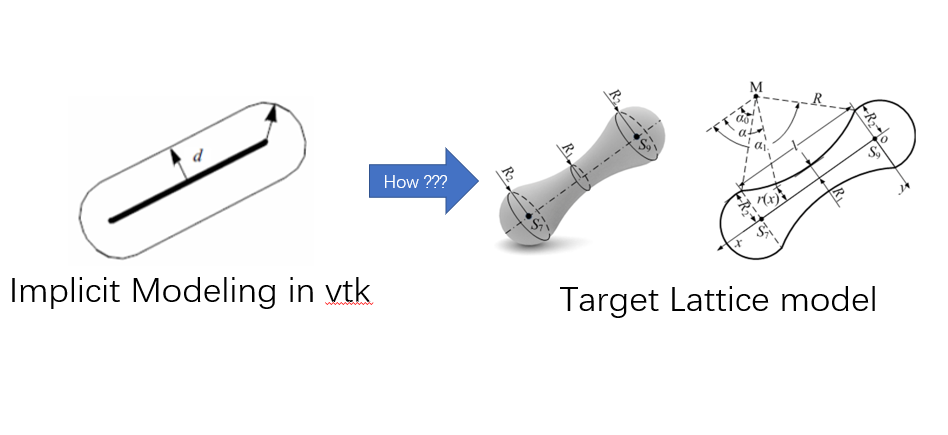# How do I build special lattice model with implicit modeling?

In VTK, is there any way to generate a lattice model similar to the one shown below by either implicit modeling or Level-set? If I switch to a lattice model like the one below, how do I build with implicit modeling?1 Like

What’s the equation used for the object on the left?

Thank you for your reply. For the left figure, I use the vtkImplicitModeller class, which automatically generates offset distance fields around points, lines, and polygons. I don’t know what the equation is or how it’s implemented, but it does implement equidistant offset. Do you have any solutions to this problem? Or do you know how to construct a distance field based on points, lines, and polygons?